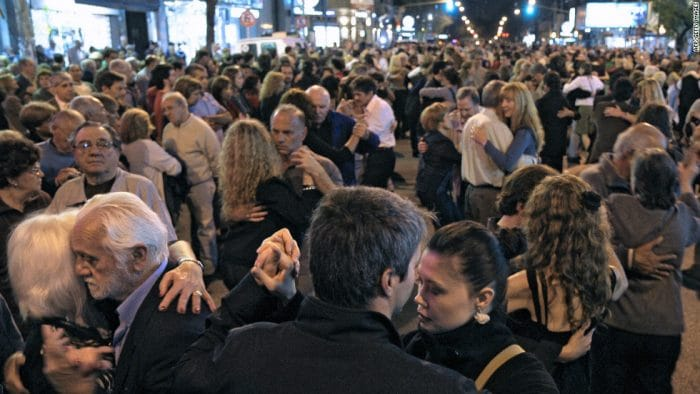# I found the secret, the key to the vault

Q. We will have 600 people at a conference.  How many possible two-person pairs does that allow?

A. In order to solve this problem, let’s solve some easier problems first.

Let’s have all 600 people line up in a row. How many ways are there to line up 600 people? Well, first we have to choose the first person. There are 600 choices for that first person, that is, any of those 600 could go first. Then, who goes second? We have 599 people left. So, to figure out how many ways we can choose the first and second people in a line of 600 people, we calculate$600 \times 599$.

Now if we continue this logic through all 600 people, choosing the first, the second, the third and so on, we have$600 \times 599 \times 598 \times ... \times 2 \times 1$ ways to line up 600 people in a row.

In other words, there are:$n!$

ways to line up n people in a row.

But that’s not the right answer to our original problem. Let’s try to get that answer closer to the original answer we wanted. Let’s say we divided each of those$n!$ rows of people evenly into pairs, taking them each two by two in order of the row. In that case, it wouldn’t matter if the first pair contained Alice or Bob, or Bob and Alice. Within a single pair, we don’t care what the ordering of the people in that pair is.

For 600 people, there are$\frac{600}{2}$ pairs or 300 pairs. So, the number of ways those pairs might have swapped the first for the second is:$2^{(n/2)}$

or$2^{300}$

Therefore, the number of ways to order 600 people, ignoring the ways to merely swap a pair of people, is:$\frac{n!}{2^{(n/2)}}$

We’ve only got one more step to get an answer. Notice that while we’ve taken care of the case where a single pair of people are swapped, we haven’t taken care of the case where a pair of people is swapped with another pair of people. In other words, we don’t care whether it’s Alice and Bob followed by Carol and Dan, or if it’s Carol and Dan followed by Alice and Bob. So, we need to also divide by the number of ways to order 300 pairs of people. As we know from above, there are$300!$ ways to order 300 things. To ignore swapping pairs of n things, we need to divide by:$(\frac{n}{2})!$

So let’s take a look at our final formula, which takes into account the ways we can choose n people, ignoring a swapped pair of two people, and also ignoring swapped pairs of people:$\frac{n!}{2^{(n/2)}(\frac{n}{2})!}$

Let’s plug in$600$ for$n$:$\frac{600!}{2^{(600/2)}(\frac{600}{2})!}$

You can calculate this answer yourself if you use this online calculator and type in this formula:

factorial(600)/((2^300)*factorial(300))

by